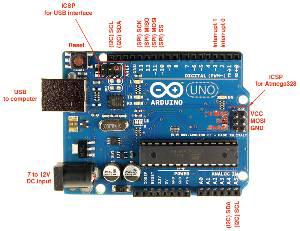Conversion of an old pinball playfield to an Arduino-driven, fully working pinball machine.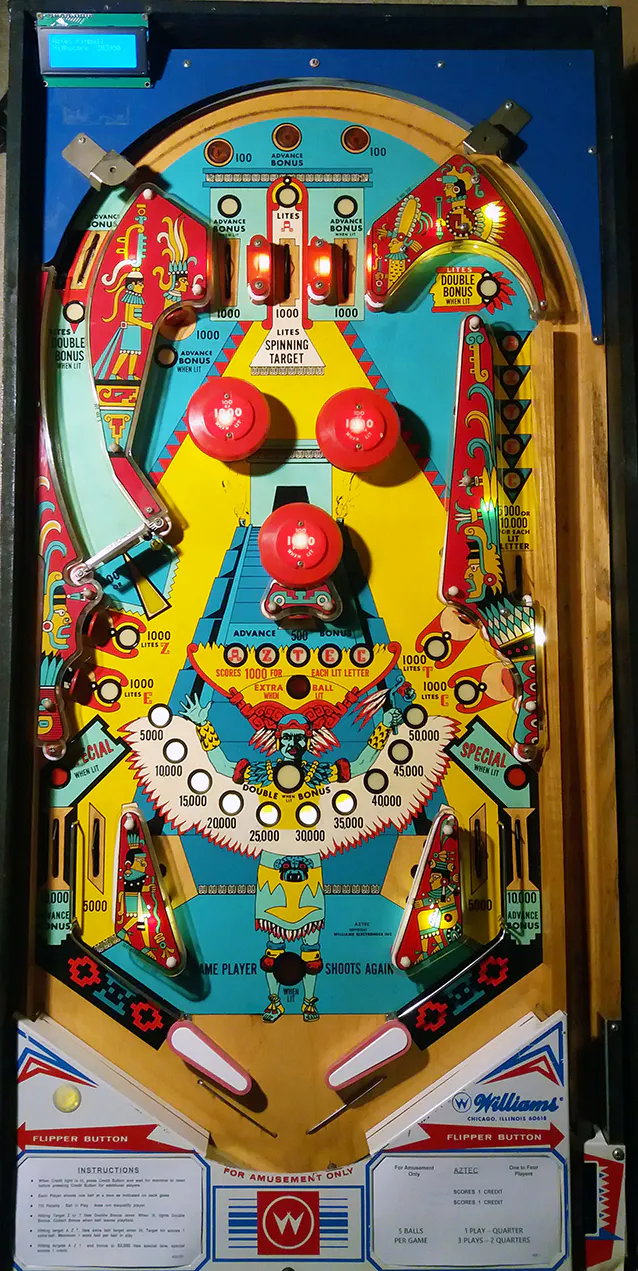## Things used in this project

### Hardware components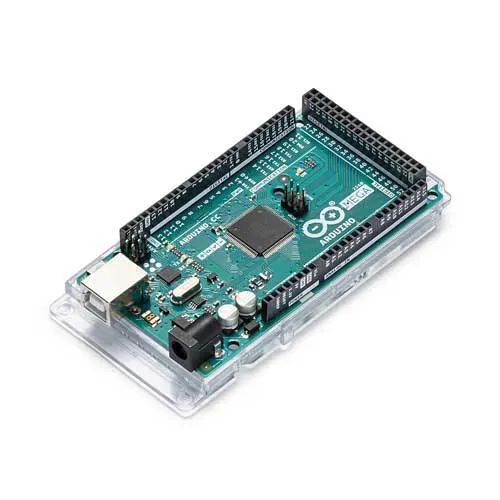Arduino Mega 2560
×1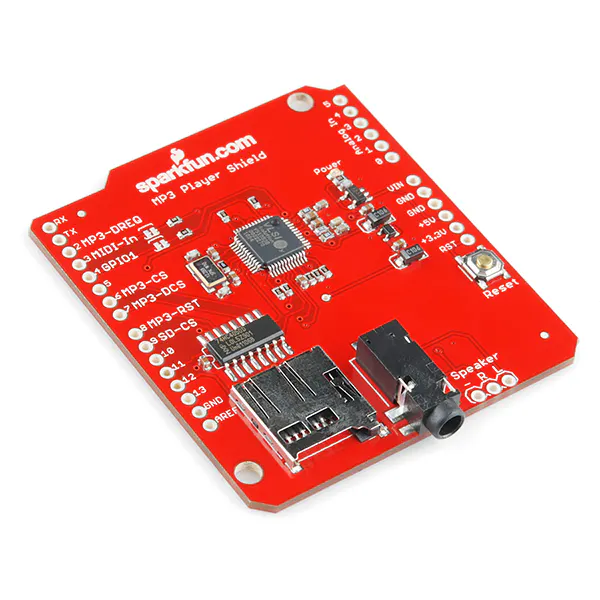SparkFun MP3 Player Shield
×1
 IIC/I2C 1602 Lcd-display-modul
×1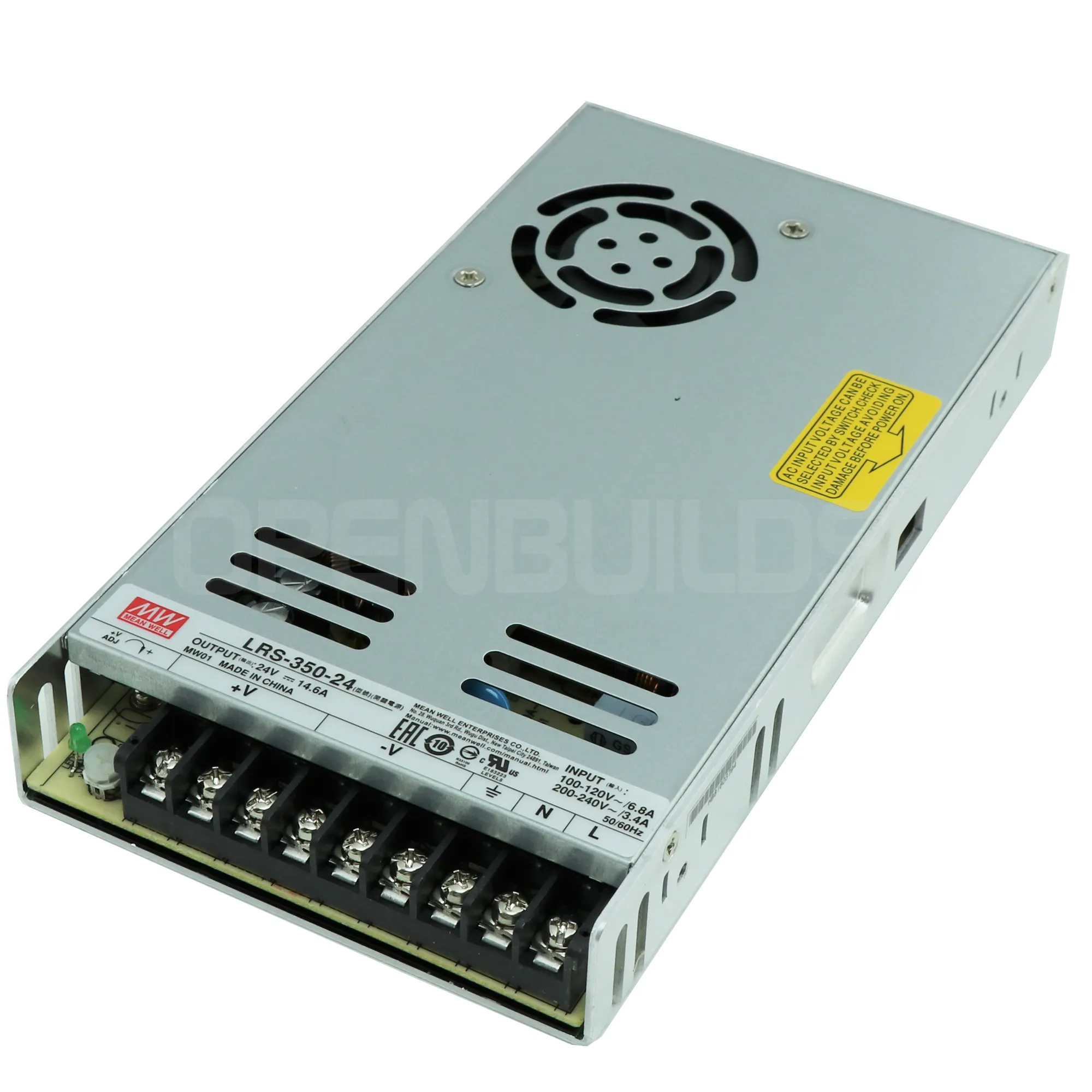OpenBuilds 24V/14.6A Power Supply
×1
 Push Buttons with microswitches
×1
 IRL540N MOSFET
×1

## Code

### Arduino controlled Pinball Machine Code

C/C++
Allows the Arduino to control the game play of a home made pinball machine. Controls lights, sensors, voltage to coils, scoring, etc.
```#include <Wire.h>
#include <LiquidCrystal_I2C.h>

#include <SPI.h>
#include <SdFat.h>
#include <FreeStack.h>
//and the MP3 Shield Library
#include <SFEMP3Shield.h>
#include <EEPROMex.h>
#include <Arduino.h>

SdFat sd;
SFEMP3Shield MP3player;

LiquidCrystal_I2C lcd(0x3f, 2, 1, 0, 4, 5, 6, 7, 3, POSITIVE);  // Set the LCD I2C address

//Variables
int Balls = 1;  //Number of Balls
int MaxBalls = 5; //Number of Maxballs
int Lapscountertemp = 0;  //Testing
int Resetcounter =0;  //Reset at Loss of Ball
long Score = 0;  //Total Score

long Highscore;
long Bonus = 0;  //Bonus
int DoubleBonus = 0;
long DoubleBonuscounter = 0;  //Counter for 2xBonus
int Arrowcounter = 0;
int Arrowbonus = 0;
int DoubleBonus_Lit = 0;
int Milli = 150; //Delay 1
int MilliRoll = 100; //Delay 2
int Milli_Bumper = 40; //Delay for Bumpers
int AZTEC_A = 0;
int AZTEC_Z = 0;
int AZTEC_T = 0;
int AZTEC_E = 0;
int AZTEC_C = 0;
int Ball_Bonus = 0;
int Kickout_Bonus =0;
int Target_Middle = 0;
int Target_Points = 0;
volatile int AttractMode = 0;
int Attractcounter1 = 0;
int Attractcounter2 = 0;
int Attractcounter3 = 0;
int Attractcounter4 = 0;
int Attractdelay1 = 800;
int Attractdelay2 = 400;
int Startplay_A = 0;
int Startplay_ACTIVE = 0;

int RolloverMitte_int_last = LOW;
int kickoutreset = 0;

int  Target_Z_last= LOW;
int  Target_T_last= LOW;
int  Target_E_last= LOW;
int  Target_C_last= LOW;
int  Target_Middle_last= LOW;
int  Target_Points_last= LOW;
int  Ramp_last= LOW;

int SwitchtopMitte_last = LOW;

//Mosfet Variables
const long interval = 40;           // interval at which to trigger solenoids (milliseconds)
unsigned long  Solenoid_TimeTemp;
unsigned long  Solenoid_FireTime;
unsigned long previousMillis = 0;
unsigned long currentMillis = 0;
int MosfetState = LOW;

int Kickout_Trigger = 0;
int BumperRechts_Trigger = 0;
int BumperUnten_Trigger = 0;

//PINS
//int Startplay = 19;
int RolloverMitte = 23;

int Kickout = 24;
int Bumper_Rechts = 26;
int Bumper_Unten = 27;

int Target_Z = 28;
int Target_T = 29;
int Target_E = 30;
int Target_C = 31;
int Target_M = 32;
int Target_P = 33;

int SwitchtopMitte = 35;
int Release = 36;

int Spinner = 37;
int Ramp = 38;

const int solenoidPin_Kickout = 44;
const int solenoidPin_BumperRechts = 46;

//50,51,52 Display
const int solenoidPin_Release = 49;
const int solenoidPin_BumperUnten = 48;

//Lights

char Light_A = A0;    //x2
char Light_Z = A1;    //x2
char Light_T = A2;    //x2
char Light_E = A3;    //x2
char Light_C = A4;    //x2
char Light_Ball_Bonus = A5;
int Light_A_Target = A6;
int Light_Z_Target = A7;
int Light_T_Target = A8;
int Light_E_Target = A9;
int Light_C_Target = A10;
int Light_Arrow = A11;
int Light_Double_Bonus_Lit = A12;   //x2
int Light_Double_Bonus = A14;  //x1

int Light__Bonus_1 = 14;
int Light__Bonus_2 = 15;
int Light__Bonus_3 = 16;
int Light__Bonus_4 = 17;
int Light__Bonus_5 = 41;//18
int Light__Bonus_6 = 19;
int Light__Bonus_7 = 40;
int Light__Bonus_8 = A15;
int Light__Bonus_9 = 5;
int Light__Bonus_10 = 47;
//mp3 shield 2,2,3,6,7,8,9

//-A15

//SOUNDS

//1 Bumper
//2 Slingshots
//3 Spinner
//4 Target
//5 Kickoutlane
//6 Ball lost
//7 Game Over

void setup() {
// put your setup code here, to run once:

lcd.begin(16,4);   // initialize the lcd for 16 chars 4 lines, turn on backlight
lcd.clear();
lcd.print("Aztec Pinball");

//MP3

sd.begin(SD_SEL, SPI_FULL_SPEED);
MP3player.begin();
//

//Variables

// Inputs

pinMode(RolloverMitte,INPUT);  //Rollover Mid
pinMode(Target_Z,INPUT);  //Target Z
pinMode(Target_T,INPUT);  //Target T
pinMode(Target_E,INPUT);  //Target E
pinMode(Target_C,INPUT);  //Target C
pinMode(Target_M,INPUT);  //Target M
pinMode(Target_P,INPUT);  //Target P

pinMode(Release,INPUT);  //Release
pinMode(Kickout,INPUT);  //Kickout
pinMode(Bumper_Rechts,INPUT);
pinMode(Bumper_Unten,INPUT);

pinMode(SwitchtopMitte,INPUT);
pinMode(Spinner,INPUT);
pinMode(Ramp,INPUT);

//pinMode(Startplay,INPUT);

//Outputs
pinMode(solenoidPin_Release, OUTPUT);
pinMode(solenoidPin_Kickout, OUTPUT);
pinMode(solenoidPin_BumperRechts, OUTPUT);
pinMode(solenoidPin_BumperUnten, OUTPUT);

pinMode(Light_A, OUTPUT);
pinMode(Light_Z, OUTPUT);
pinMode(Light_T, OUTPUT);
pinMode(Light_E, OUTPUT);
pinMode(Light_C, OUTPUT);
pinMode(Light_Ball_Bonus, OUTPUT);
pinMode(Light_Arrow, OUTPUT);

pinMode(Light_A_Target, OUTPUT);
pinMode(Light_Z_Target, OUTPUT);
pinMode(Light_T_Target, OUTPUT);
pinMode(Light_E_Target, OUTPUT);
pinMode(Light_C_Target, OUTPUT);

pinMode(Light_Double_Bonus_Lit, OUTPUT);
pinMode(Light_Double_Bonus, OUTPUT);

pinMode(Light__Bonus_1, OUTPUT);
pinMode(Light__Bonus_2, OUTPUT);
pinMode(Light__Bonus_3, OUTPUT);
pinMode(Light__Bonus_4, OUTPUT);
pinMode(Light__Bonus_5, OUTPUT);
pinMode(Light__Bonus_6, OUTPUT);
pinMode(Light__Bonus_7, OUTPUT);
pinMode(Light__Bonus_8, OUTPUT);
pinMode(Light__Bonus_9, OUTPUT);
pinMode(Light__Bonus_10, OUTPUT);
/////////

digitalWrite(Light_A, LOW);
digitalWrite(Light_Z, LOW);
digitalWrite(Light_T, LOW);
digitalWrite(Light_E, LOW);
digitalWrite(Light_C, LOW);
digitalWrite(Light_Ball_Bonus, LOW);
digitalWrite(Light_Arrow, LOW);

digitalWrite(Light_Double_Bonus_Lit, LOW);
digitalWrite(Light_Double_Bonus, LOW);

digitalWrite(Light_A_Target, HIGH);
digitalWrite(Light_Z_Target, HIGH);
digitalWrite(Light_T_Target, HIGH);
digitalWrite(Light_E_Target, HIGH);
digitalWrite(Light_C_Target, HIGH);

digitalWrite(Light__Bonus_1, LOW);
digitalWrite(Light__Bonus_2, LOW);
digitalWrite(Light__Bonus_3, LOW);
digitalWrite(Light__Bonus_4, LOW);
digitalWrite(Light__Bonus_5, LOW);
digitalWrite(Light__Bonus_6, LOW);
digitalWrite(Light__Bonus_7, LOW);
digitalWrite(Light__Bonus_8, LOW);
digitalWrite(Light__Bonus_9, LOW);
digitalWrite(Light__Bonus_10, LOW);

}  //END SETUP

//////////////////////////////////////////////////////////////////////////////////////////////////////////MAIN
void loop() {
// put your main code here, to run repeatedly:

EIFR = bit (INTF5);
attachInterrupt(5, interruptstart, RISING);

if (AttractMode == 0) {

ATTRACT();
} //END ATTRACT

if (AttractMode == 1)  {

if (Startplay_ACTIVE == 0){

Startplay_ACTIVE = 1;
MP3player.stopTrack();
MP3player.playMP3("009.mp3");
ResetGame();
Kickout_ball();
}

unsigned long currentMillis = millis();       //Starting Time
detectCollision();                              //Collision and Switch detection
control_lights();                              //Lights
check_conditions();                            //Checks for Bonuses

//Timed Soneloid Triggers running in Main Loop

//MOSFET !

int difference = currentMillis - previousMillis;

if ((difference >= interval)and (BumperLinks_Trigger == 1)) {
previousMillis = currentMillis;
}
if ((difference >= interval)and (BumperRechts_Trigger == 1)) {
previousMillis = currentMillis;
BumperRechts_Trigger = 0;
digitalWrite(solenoidPin_BumperRechts, LOW);
}
if ((difference >= interval)and (BumperUnten_Trigger == 1)) {
previousMillis = currentMillis;
BumperUnten_Trigger = 0;
digitalWrite(solenoidPin_BumperUnten, LOW);
}

}
}
//////////////////////////////////////////////////////////////////////////////////////////////////////////MAIN ENDE
void interruptstart(){      //  ISR!

AttractMode = 1;
detachInterrupt(5);
}

void ATTRACT (){      //Bad, lazy code !!
lcd.clear();
lcd.print("Aztec Pinball");
lcd.setCursor(0,1);
lcd.print("Highscore  ");
lcd.print(Highscore);

while (AttractMode == 0) {

lcd.clear();
lcd.print("Aztec Pinball");
lcd.setCursor(0,1);
lcd.print("Highscore  ");
lcd.print(Highscore);

Attractcounter1 = 0;
Attractcounter2 = 0;
Attractcounter3 = 0;
Attractcounter4 = 0;
AttractMode = 0;

digitalWrite(Light_A_Target, LOW);
digitalWrite(Light_Z_Target, LOW);
digitalWrite(Light_T_Target, LOW);
digitalWrite(Light_E_Target, LOW);
digitalWrite(Light_C_Target, LOW);
digitalWrite(Light_A, LOW);
digitalWrite(Light_Z, LOW);
digitalWrite(Light_T, LOW);
digitalWrite(Light_E, LOW);
digitalWrite(Light_C, LOW);
digitalWrite(Light_Ball_Bonus, LOW);
digitalWrite(Light_Arrow, LOW);
digitalWrite(Light_Double_Bonus_Lit, LOW);
digitalWrite(Light_Double_Bonus, LOW);
digitalWrite(Light__Bonus_1, LOW);
digitalWrite(Light__Bonus_2, LOW);
digitalWrite(Light__Bonus_3, LOW);
digitalWrite(Light__Bonus_4, LOW);
digitalWrite(Light__Bonus_5, LOW);
digitalWrite(Light__Bonus_6, LOW);
digitalWrite(Light__Bonus_7, LOW);
digitalWrite(Light__Bonus_8, LOW);
digitalWrite(Light__Bonus_9, LOW);
digitalWrite(Light__Bonus_10, LOW);

digitalWrite(Light__Bonus_1, HIGH);
delay(Attractdelay1);if (AttractMode == 1)  {break;};
digitalWrite(Light__Bonus_2, HIGH);
delay(Attractdelay1);if (AttractMode == 1)  {break;};
digitalWrite(Light__Bonus_3, HIGH);
delay(Attractdelay1);if (AttractMode == 1)  {break;};
digitalWrite(Light__Bonus_4, HIGH);
delay(Attractdelay1);if (AttractMode == 1)  {break;};
digitalWrite(Light__Bonus_5, HIGH);
delay(Attractdelay1);if (AttractMode == 1)  {break;};
digitalWrite(Light__Bonus_6, HIGH);
delay(Attractdelay1);if (AttractMode == 1)  {break;};
digitalWrite(Light__Bonus_7, HIGH);
delay(Attractdelay1);if (AttractMode == 1)  {break;};
digitalWrite(Light__Bonus_8, HIGH);
delay(Attractdelay1);if (AttractMode == 1)  {break;};
digitalWrite(Light__Bonus_9, HIGH);
delay(Attractdelay1);if (AttractMode == 1)  {break;};
digitalWrite(Light__Bonus_10, HIGH);
delay(Attractdelay1);if (AttractMode == 1)  {break;};
while (Attractcounter1 <4){
digitalWrite(Light__Bonus_1, LOW);
digitalWrite(Light__Bonus_2, LOW);
digitalWrite(Light__Bonus_3, LOW);
digitalWrite(Light__Bonus_4, LOW);
digitalWrite(Light__Bonus_5, LOW);
digitalWrite(Light__Bonus_6, LOW);
digitalWrite(Light__Bonus_7, LOW);
digitalWrite(Light__Bonus_8, LOW);
digitalWrite(Light__Bonus_9, LOW);
digitalWrite(Light__Bonus_10, LOW);
delay(Attractdelay2);if (AttractMode == 1)  {break;};
digitalWrite(Light__Bonus_1, HIGH);
digitalWrite(Light__Bonus_2, HIGH);
digitalWrite(Light__Bonus_3, HIGH);
digitalWrite(Light__Bonus_4, HIGH);
digitalWrite(Light__Bonus_5, HIGH);
digitalWrite(Light__Bonus_6, HIGH);
digitalWrite(Light__Bonus_7, HIGH);
digitalWrite(Light__Bonus_8, HIGH);
digitalWrite(Light__Bonus_9, HIGH);
digitalWrite(Light__Bonus_10, HIGH);
delay(Attractdelay2);if (AttractMode == 1)  {break;};
Attractcounter1 +=1;
}

digitalWrite(Light_A, HIGH);
digitalWrite(Light_A_Target, HIGH);
delay(Attractdelay1);if (AttractMode == 1)  {break;};
digitalWrite(Light_Z, HIGH);
digitalWrite(Light_Z_Target, HIGH);
delay(Attractdelay1);if (AttractMode == 1)  {break;};
digitalWrite(Light_T, HIGH);
digitalWrite(Light_T_Target, HIGH);
delay(Attractdelay1);if (AttractMode == 1)  {break;};
digitalWrite(Light_E, HIGH);
digitalWrite(Light_E_Target, HIGH);
delay(Attractdelay1);if (AttractMode == 1)  {break;};
digitalWrite(Light_C, HIGH);
digitalWrite(Light_C_Target, HIGH);
delay(Attractdelay1);if (AttractMode == 1)  {break;};

while (Attractcounter2 <4){
digitalWrite(Light_A, LOW);
digitalWrite(Light_A_Target, LOW);
digitalWrite(Light_Z, LOW);
digitalWrite(Light_Z_Target, LOW);
digitalWrite(Light_T, LOW);
digitalWrite(Light_T_Target, LOW);
digitalWrite(Light_E, LOW);
digitalWrite(Light_E_Target, LOW);
digitalWrite(Light_C, LOW);
digitalWrite(Light_C_Target, LOW);
delay(Attractdelay2);if (AttractMode == 1)  {break;};
digitalWrite(Light_A, HIGH);
digitalWrite(Light_A_Target, HIGH);
digitalWrite(Light_Z, HIGH);
digitalWrite(Light_Z_Target, HIGH);
digitalWrite(Light_T, HIGH);
digitalWrite(Light_T_Target, HIGH);
digitalWrite(Light_E, HIGH);
digitalWrite(Light_E_Target, HIGH);
digitalWrite(Light_C, HIGH);
digitalWrite(Light_C_Target, HIGH);
delay(Attractdelay2);if (AttractMode == 1)  {break;};
Attractcounter2 +=1;
}

digitalWrite(Light_Ball_Bonus, HIGH);
delay(Attractdelay1);if (AttractMode == 1)  {break;};
digitalWrite(Light_Arrow, HIGH);
delay(Attractdelay1);if (AttractMode == 1)  {break;};
digitalWrite(Light_Double_Bonus_Lit, HIGH);
delay(Attractdelay1);if (AttractMode == 1)  {break;};
digitalWrite(Light_Double_Bonus, HIGH);
delay(Attractdelay1);if (AttractMode == 1)  {break;};
delay(Attractdelay1);if (AttractMode == 1)  {break;};
while (Attractcounter3 <4){
digitalWrite(Light_Ball_Bonus, LOW);
digitalWrite(Light_Arrow, LOW);
digitalWrite(Light_Double_Bonus_Lit, LOW);
digitalWrite(Light_Double_Bonus, LOW);
delay(Attractdelay2);if (AttractMode == 1)  {break;};
digitalWrite(Light_Ball_Bonus, HIGH);
digitalWrite(Light_Arrow, HIGH);
digitalWrite(Light_Double_Bonus_Lit, HIGH);
digitalWrite(Light_Double_Bonus, HIGH);
delay(Attractdelay2);if (AttractMode == 1)  {break;};
Attractcounter3 +=1;
}
delay(2000);if (AttractMode == 1)  {break;};

digitalWrite(Light__Bonus_1, LOW);
digitalWrite(Light_Ball_Bonus, LOW);
delay(Attractdelay1);if (AttractMode == 1)  {break;};
digitalWrite(Light__Bonus_2, LOW);
digitalWrite(Light_C, LOW);
digitalWrite(Light_Z_Target, LOW);
delay(Attractdelay1);if (AttractMode == 1)  {break;};
digitalWrite(Light__Bonus_3, LOW);
delay(Attractdelay1);if (AttractMode == 1)  {break;};
digitalWrite(Light__Bonus_4, LOW);
digitalWrite(Light_E, LOW);
delay(Attractdelay1);if (AttractMode == 1)  {break;};
digitalWrite(Light_Arrow, LOW);
digitalWrite(Light__Bonus_5, LOW);
digitalWrite(Light_T_Target, LOW);
delay(Attractdelay1);if (AttractMode == 1)  {break;};
digitalWrite(Light__Bonus_6,LOW);
digitalWrite(Light_T, LOW);
delay(Attractdelay1);if (AttractMode == 1)  {break;};
digitalWrite(Light__Bonus_7, LOW);
digitalWrite(Light_E_Target, LOW);
delay(Attractdelay1);if (AttractMode == 1)  {break;};
digitalWrite(Light_Double_Bonus_Lit, LOW);
digitalWrite(Light__Bonus_8, LOW);
digitalWrite(Light_Z, LOW);
delay(Attractdelay1);if (AttractMode == 1)  {break;};
digitalWrite(Light__Bonus_9, LOW);
digitalWrite(Light_C_Target, LOW);
digitalWrite(Light_Double_Bonus, LOW);
delay(Attractdelay1);if (AttractMode == 1)  {break;};
digitalWrite(Light__Bonus_10, LOW);
digitalWrite(Light_A, LOW);
digitalWrite(Light_A_Target, LOW);
delay(Attractdelay1);if (AttractMode == 1)  {break;};

}
}

void check_conditions(){
if ((AZTEC_A ==1) && (AZTEC_Z ==1) && (AZTEC_T ==1) && (AZTEC_E ==1) && (AZTEC_C ==1 && (Resetcounter ==0))){
Ball_Bonus =1;
DoubleBonus =1;
Resetcounter = 1;
}

if (DoubleBonuscounter >50) {
DoubleBonus =1;
}

}
else if (AdvanceBonus == 1) {
}
}
}

void detectCollision(){

int RolloverMitte_int = digitalRead(RolloverMitte);  //Variable for Rollover Switches

int SwitchtopMitte_int = digitalRead(SwitchtopMitte);  //Variable for Rollover Switches
int Ramp_int = digitalRead(Ramp);  //Variable for Ramp
int Spinner_int = digitalRead(Spinner);  //Variable for Spinner

int Target_Z_int = digitalRead(Target_Z);  //Variable for Target Z
int Target_T_int = digitalRead(Target_T);  //Variable for Target T
int Target_E_int = digitalRead(Target_E);  //Variable for Target E
int Target_C_int = digitalRead(Target_C);  //Variable for Target C
int Target_M_int = digitalRead(Target_M);  //Variable for Target M
int Target_P_int = digitalRead(Target_P);  //Variable for Target P

//Rollovers Links und Rechts und Mitte

Score100f();
MP3player.stopTrack();
MP3player.playMP3("001.mp3");
}

else if ((RolloverLinks_Rechts_int == LOW) && (RolloverLinks_Rechts_int_last == HIGH)  ){    //Rollover released
delay (MilliRoll);
}

if ((RolloverMitte_int == HIGH) && (RolloverMitte_int_last == LOW)  ){      //Rollover pressed

Bonusf();
RolloverMitte_int_last = HIGH;
MP3player.stopTrack();
MP3player.playMP3("001.mp3");
}

else if ((RolloverMitte_int == LOW) && (RolloverMitte_int_last == HIGH)  ){ //Rollover released
RolloverMitte_int_last = LOW;
delay (MilliRoll);
}
//Switches Left, right and mid

Arrowbonus =0;      //deacitvate Spinner Bonus

Score1000f();
}
else if (AdvanceBonus == 1) {
Score1000f();
Bonusf();
}

//MP3player.stopTrack();
// MP3player.playMP3("001.mp3");
}

else if ((SwitchtopLinks_Rechts_int == LOW) && (SwitchtopLinks_Rechts_last == HIGH)  ){    //Rollover released
delay (MilliRoll);
}

if ((SwitchtopMitte_int == HIGH) && (SwitchtopMitte_last == LOW)  ){      //Rollover pressed
Arrowbonus = 1;        //acitvate Spinner Bonus
AZTEC_Af();
SwitchtopMitte_last = HIGH;
//MP3player.stopTrack();
//MP3player.playMP3("001.mp3");
}

else if ((SwitchtopMitte_int == LOW) && (SwitchtopMitte_last == HIGH)  ){    //Rollover released
SwitchtopMitte_last = LOW;
delay (MilliRoll);
}

//Slingshots

Score150f();
MP3player.stopTrack();
MP3player.playMP3("002.mp3");
DoubleBonuscounter +=1;
//lcd.setCursor(4,3);
//lcd.print("Slingshots");
}

else if ((Slingshots_Links_Rechts_int == LOW) && (Slingshots_Links_Rechts_last == HIGH)  ){    //Rollover released
delay (MilliRoll);
}

//Outlanes

Bonusf();
Score10000f();
//lcd.setCursor(4,3);
//lcd.print("Outlane");
MP3player.stopTrack();
MP3player.playMP3("001.mp3");
}

else if ((Outlanes_Links_Rechts_int == LOW) && (Outlanes_Links_Rechts_last == HIGH)  ){    //Rollover released
delay (MilliRoll);
}

//Sidelanes

Score5000f();
//lcd.setCursor(4,3);
//lcd.print("Sidelane");
MP3player.stopTrack();
MP3player.playMP3("001.mp3");
}

else if ((Sidelanes_Links_Rechts_int == LOW) && (Sidelanes_Links_Rechts_last == HIGH)  ){    //Rollover released
delay (MilliRoll);
}

//RAMP
if ((Ramp_int == HIGH) && (Ramp_last == LOW)  ){      //RAMP Pressed

Rampf();
Ramp_last = HIGH;
}

else if ((Ramp_int == LOW) && (Ramp_last == HIGH)  ){    //Ramp released
Ramp_last = LOW;
delay (MilliRoll);
}

// TARGETS

if ((Target_Z_int == HIGH) && (Target_Z_last == LOW)  ){      //Target hit
Target_Z_last = HIGH;

AZTEC_Z = 1;
Score = Score +1000;
//lcd.setCursor(4,3);
//lcd.print("Z");
MP3player.stopTrack();
MP3player.playMP3("004.mp3");

delay (MilliRoll);
}

else if ((Target_Z_int == LOW) && (Target_Z_last == HIGH)  ){ //Target released
Target_Z_last = LOW;
delay (MilliRoll);
}

if ((Target_T_int == HIGH) && (Target_T_last == LOW)  ){      //Target hit
Target_T_last = HIGH;

AZTEC_T = 1;
Score = Score +1000;
//lcd.setCursor(4,3);
//lcd.print("T");
MP3player.stopTrack();
MP3player.playMP3("004.mp3");

delay (MilliRoll);
}

else if ((Target_T_int == LOW) && (Target_T_last == HIGH)  ){ //Target released
Target_T_last = LOW;
delay (MilliRoll);
}

if ((Target_E_int == HIGH) && (Target_E_last == LOW)  ){      //Target hit
Target_E_last = HIGH;

AZTEC_E = 1;
Score = Score +1000;
//lcd.setCursor(4,3);
//lcd.print("E");
MP3player.stopTrack();
MP3player.playMP3("004.mp3");

delay (MilliRoll);
}

else if ((Target_E_int == LOW) && (Target_E_last == HIGH)  ){ //Target released
Target_E_last = LOW;
delay (MilliRoll);
}

if ((Target_C_int == HIGH) && (Target_C_last == LOW)  ){      //Target hit
Target_C_last = HIGH;

AZTEC_C = 1;
Score = Score +1000;
//lcd.setCursor(4,3);
//lcd.print("C");
MP3player.stopTrack();
MP3player.playMP3("004.mp3");

delay (MilliRoll);
}

else if ((Target_C_int == LOW) && (Target_C_last == HIGH)  ){ //Target released
Target_C_last = LOW;
delay (MilliRoll);
}

if ((Target_M_int == HIGH) && (Target_Middle_last == LOW)  ){      //Target hit
Target_Middle_last = HIGH;

Target_Middle = 1;
Score = Score +500;
if (Ball_Bonus ==1){
MaxBalls +=1;
Ball_Bonus = 0;
digitalWrite(Light_Ball_Bonus, LOW);
}

MP3player.stopTrack();
MP3player.playMP3("004.mp3");
Bonusf();

delay (MilliRoll);
}

else if ((Target_M_int == LOW) && (Target_Middle_last == HIGH)  ){ //Target released
Target_Middle_last = LOW;
delay (MilliRoll);
}

if ((Target_P_int == HIGH) && (Target_Points_last == LOW)  ){      //Target hit
Target_Points_last = HIGH;

Target_Points += 1;
//MP3player.stopTrack();
//MP3player.playMP3("004.mp3");

Score1000f();
}
else if (AdvanceBonus == 1) {
Score1000f();
Bonusf();
}

delay (45); //shorter because of Bumpers
}

else if ((Target_P_int == LOW) && (Target_Points_last == HIGH)  ){ //Target released
Target_Points_last = LOW;
delay (MilliRoll);
}

//SPINNER

Spinnerf();

}

//Ball Release
Releasef();

}

//Kickout
if ((digitalRead(Kickout) == HIGH)&& (kickoutreset ==0) ){
kickoutreset = 1;
Kickoutf();

}

//Bumpers

BumperLf();

}

BumperRf();

}

BumperUf();

}
}  // ENDE Collision Detection

//Lights

void control_lights(){

if (AZTEC_A ==1){
digitalWrite(Light_A, HIGH);
digitalWrite(Light_A_Target, LOW);
}
if (AZTEC_Z ==1){
digitalWrite(Light_Z, HIGH);
digitalWrite(Light_Z_Target, LOW);
}
if (AZTEC_T ==1){
digitalWrite(Light_T, HIGH);
digitalWrite(Light_T_Target, LOW);
}
if (AZTEC_E ==1){
digitalWrite(Light_E, HIGH);
digitalWrite(Light_E_Target, LOW);
}
if (AZTEC_C ==1){
digitalWrite(Light_C, HIGH);
digitalWrite(Light_C_Target, LOW);
}
if (Ball_Bonus ==1){
digitalWrite(Light_Ball_Bonus, HIGH);
}

if (DoubleBonus == 1){

digitalWrite(Light_Double_Bonus, HIGH);
}

else if (DoubleBonus == 0){
digitalWrite(Light_Double_Bonus, LOW);
}

//###########
if (DoubleBonus_Lit ==1){

digitalWrite(Light_Double_Bonus_Lit, HIGH);
}

else if (DoubleBonus_Lit ==0){
digitalWrite(Light_Double_Bonus_Lit, LOW);
}

//################

if (Arrowbonus ==1){
digitalWrite(Light_Arrow, HIGH);
}

else if (Arrowbonus ==0){
digitalWrite(Light_Arrow, LOW);
}

}
else if (AdvanceBonus == 0) {
}

//Bonus Lights
//###############

if (Bonus == 5000) {
digitalWrite(Light__Bonus_1, HIGH);
}
else if (Bonus == 10000) {
digitalWrite(Light__Bonus_2, HIGH);
}

else if (Bonus == 15000) {
digitalWrite(Light__Bonus_3, HIGH);
}
else if (Bonus == 20000) {
digitalWrite(Light__Bonus_4, HIGH);
...